# A Cost Analysis for Urban Transformation Applications

Halil Ibrahim Polat*

Department of Civil Engineering and Architecture, Turkey

Received Date: April 18, 2019;  Published Date: May 13, 2019

#### Abstract

Urban transformation is a method that is used for renewal of natural disaster and earthquake risks at high levels, has not completed its social development, and has a population density exceeding the capacity. The economic aspect of the transaction, which is intended to be made outside the zoning and planning criteria, should also be taken into consideration when making conversion decisions.

In this study, a region in Turkey which is declared as risky area under the Law no 6306: The transformation of areas at risk of disaster is discussed. The urban transformation costs in the area of 4.6 hectares with a population of 1430 people are tried to be calculated in the light of the data obtained from the feasibility reports made in the region. For these costs analyzes, Appropriate Urban Transformation Model (AUTM) has been utilized.

Keywords: Urban transformation; Architecture; Planning; Urbanism; Cost

#### Introduction

Urban transformation can be defined as a comprehensive vision and action that seeks to provide a permanent solution to the economic, physical, social and environmental conditions of a region that provides for the solution of urban problems . It can be seen as a space arrangement .

The Appropriate Urban Transformation Model (AUTM)  is a mathematical model. The basic ideal of an urban transformation zone is to create planned, healthy and sustainable living spaces. In this article; a model extending from urban and social infrastructure, zoning, and cost analysis to seismicity was tried to be put forward. In this context, a literature review on the subject was made. Urban standards were discussed, numerical values were entered into the database and inputs are calculated by analyzing the data.

In this study; the data obtained from urban infrastructure, zoning and seismicity parameters have been used and it has not been given in detail considering the limited possibilities of the article. However, it is tried to calculate what kind of transformation cost is faced as a result of these data. In this article, the analysis is customized on costs.At AUTM, in summary, a set of parameters related to any field is determined and transferred to a database. By analyzing the data with the developed mathematical formulas, the decisions of the transformation could be taken. The results of the analysis of all numerical data reveal a picture that will help to interpret whether transformation is necessary. In this mathematical model, there are 4 model groups under current and appropriate status titles. A1 and A2 model group includes urban and social infrastructure data, whereas model group B1 and B2 deal with zoning data. The C model group contains cost data and the D model group constitutes the seismicity data. There is an algorithmic relationship between these model groups (Figure 1).

According to the network of relationships in Figure 1; the data A1 provides data flow to data B1, data A2 into the data B1 and C. At the same time, B1 and B2 data are transferred to group C and group D to data group B2. For the cost data of model C model, which is the main subject of this study, the parameters of model A2, B1 and B2 are determinative.

In order to carry out all these analyzes, a place which is declared as risky area has been selected in Istanbul within the framework of the Urban Transformation Act No. 6306. This place consists of 11 islands and 219 parcels. Information on all structures planned to be demolished in the area was collected . The zoning applications of the buildings that are planned to be constructed, instead of the structures to be demolished are calculated within the framework of AUTM. The area with an urban infrastructure rate of 50.90% with a population of 1430 is 4.6 hectares. The cost analysis of a risky area with a public space of 34.10%, 1.76 floor area ratio, 0.31 building coverage ratio, all the structures limited to 6 storeys is tried to be done.

#### Cost Analysis

Urban transformation sometimes triggers a physical destruction . In this context, it is the subject of architecture and planning as well as the economy. Therefore, cost analysis of the region to be transformed is extremely important. In this section, cost data is analyzed according to the AUTM spreadsheets. The parameters of primarily construction sites areas, demolition, excavation and contractor payments are shown in the following table for the calculation of these data (Table 1) .

For the aforementioned costs, approximate unit costs of 2016 building which are used in the calculation of architectural and engineering service costs of the Ministry of Environment and Urbanization are taken as the basis of approximate unit costs in the communiqué numbered 29679 .

##### Residential construction cost data

The unit cost of housing construction in 2016, III B group housing buildings (with a building height less than 21.50) is taken as 750,00 (TL/m²). As shown in the house construction cost data interaction diagram, the cost of housing construction, which belongs to cost group C, is the first factor that affects the net cost (TL) parameter from model C model (Figure 2).In the equation (1), the appropriate residential building cost is calculated as 39.117.163.26 TL by multiplying the cost of residential construction unit with appropriate gross residential building area (field data).##### Commercial construction cost data

The unit cost of trade construction in 2016 is taken as 750,00 (TL/m²) for the commercial buildings of B Group III (offices, restaurants, cafeteria, etc.). There is no dedicated zone in the project area. The cost of commercial construction that belongs to the cost group C as shown in the diagram of the commercial construction cost data interaction; it is the factor that affects the net cost (TL) parameter from the C model group (Figure 3).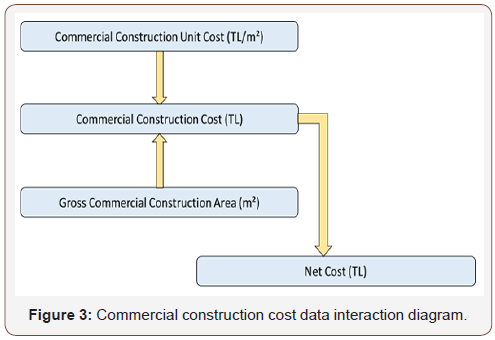In accordance with equation (2) equality, the appropriate gross commercial construction area (field data) is multiplied by commercial construction unit cost and the appropriate commercial construction cost is found as 0.00 TL. Because there is no only commercial are in the design zone.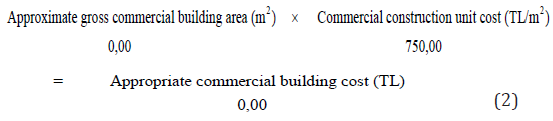##### Residential + commercial construction cost data

In 2016, the unit cost of residential + commercial construction is determined as 750,00 (TL / m²) for III B group housing + trade structures (with a building height less than 21.50m) (Figure 4).The cost of residential + commercial construction data which is owned by the group C as shown in the residential + commercial construction cost data interaction diagram is the first factor affecting the net cost (TL) parameter.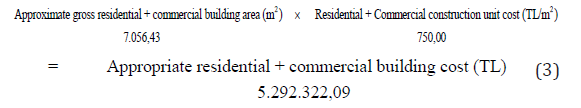With the equation (3), appropriate gross residential + commercial construction area (field data), housing + trade construction unit cost multiplied by the appropriate housing + trade construction cost and is obtained as 5.292.322,09 TL.

##### Appropriate total construction cost data

The appropriate total construction cost (residential, commercial and residential + commercial) is calculated as TL 44.409.485,35 (Figure 5).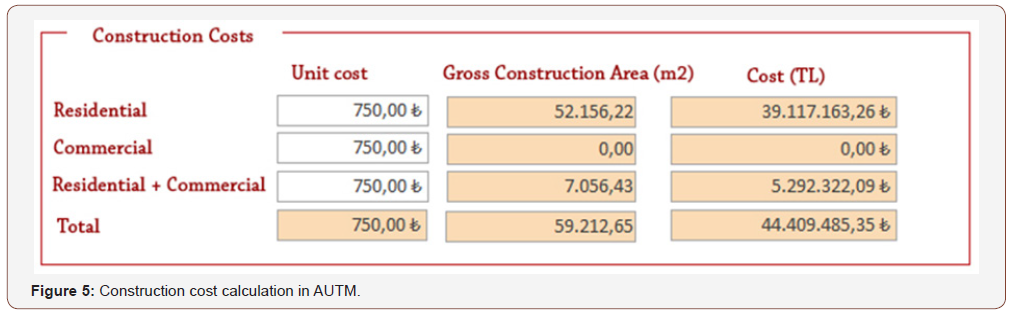##### Appropriate recreation cost data

The appropriate total recreation area is obtained by subtracting the appropriate total construction floor coverage area from the appropriate total zoning area (Figure 6).The cost of recreation (landscaping) which belongs to cost group C as shown in the recreation (landscape) cost data interaction diagram; it is one of the first-degree factors that affects net cost (TL) parameter from model group C (Table 1).According to the unit price list of the Chamber of Landscape Architects in 2016; for the project area in question, the unit cost of the 3rd class services recreation (landscaping), the creation of a grass field, field arrangement with seasonal and perennial plants, planting by shrub, planting trees, landscaping structures and environmental reinforcement elements are calculated totally as 555 m²/TL. and entered the model (Table 2) .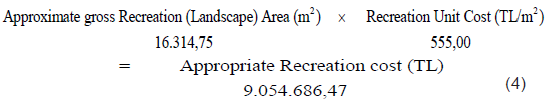Table 1: Cost data index.Table 2: Recreation (Landscaping) unit costs - 2016 year.With the help of the equation (4), the appropriate recreation (landscape) area (field data) and the recreation (landscape) unit cost are obtained.

##### Existing gross total construction volume

This data of the current situation is needed to account for the cost of demolition. It is a parameter that uses field-specific data.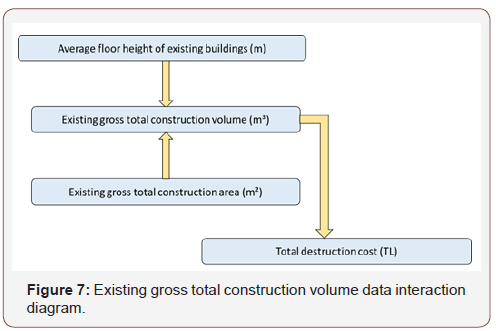Existing gross total construction volume (m3) belonging to cost group C as shown in the gross construction volume data interaction diagram, is the factor that affects the first degree the total demolition cost (TL) parameter from the C model group (Figure 7).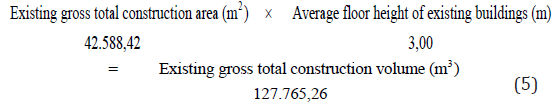With the equation (5), the existing gross total construction area (field data) was multiplied by the average floor height of 3.00m, resulting in a gross total construction volume of 127.765.26 m3.

##### Total destruction cost data

Total destruction cost data belonging to cost group C (TL) as shown in the total demolition cost data interaction diagram, is the factor that affects the first degree the net cost (TL) parameter from the C model group is (Figure 8).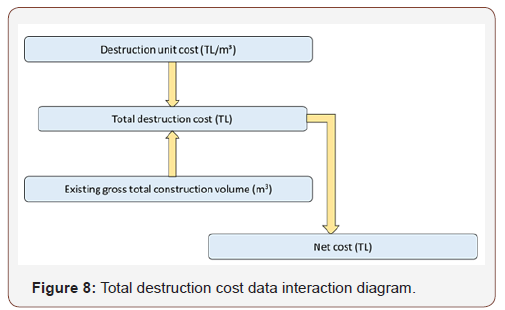From the unit price book of the Ministry of Environment and Urbanization; Exposure No: 18.180 the unit price of the demolition of mortar construction without using explosives is 21.00 TL/m3 .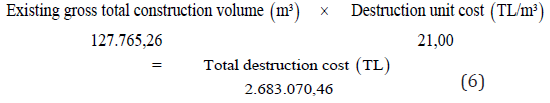With the equation (6), the existing gross total construction volume (m³) (field data) multiplied by the destruction unit cost (TL/m³) and obtained total destruction cost (TL) as 2.683.070.46 TL (Figure 9).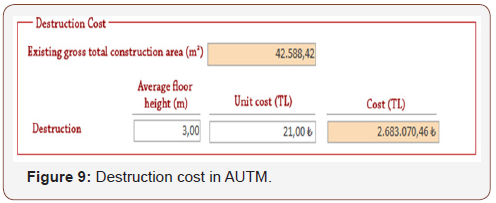##### Total soil volume based on excavation data

Total destruction cost data belonging to cost group C (TL) as shown in the total demolition cost data interaction diagram, is the factor that affects at the first degree the total excavation cost (TL) parameter from the C model group (Figure 10).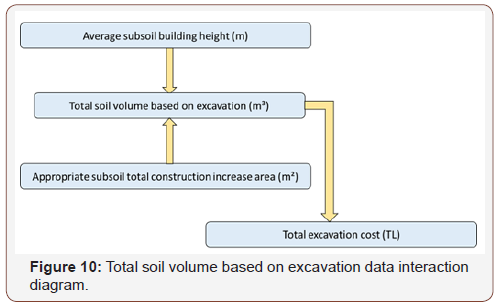According to the equation (7) the total soil volume based on excavation (m³) is found as 54.657.84 m3 by multiplying the average subsoil building height (average floor height (3.00) + foundation height (1.00) = 4.00 m.) with the appropriate subsoil total construction increase area (m²).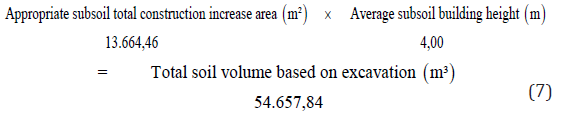##### Total excavation cost data

Total excavation cost (TL) belonging to cost group C as shown in the total excavation cost data interaction diagram; is the factor that affects at the first degree the net cost (TL) parameter from the C model group (Figure 11).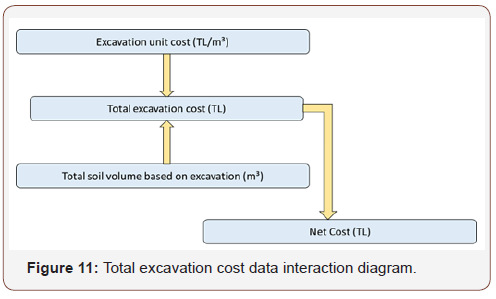Calculations have been made from the unit price book of the Ministry of Environment and Urbanization by using Pos No: 14.014/2 (Unit price 69.63 TL/m3 for soft, hard, very hard rock at every depth using hand or compressor and explosive material) .With the equation (8) total excavation cost is calculated as 3.805.824,57 TL by multiplying the total underground volume (field data) and excavation unit cost (Figure 12).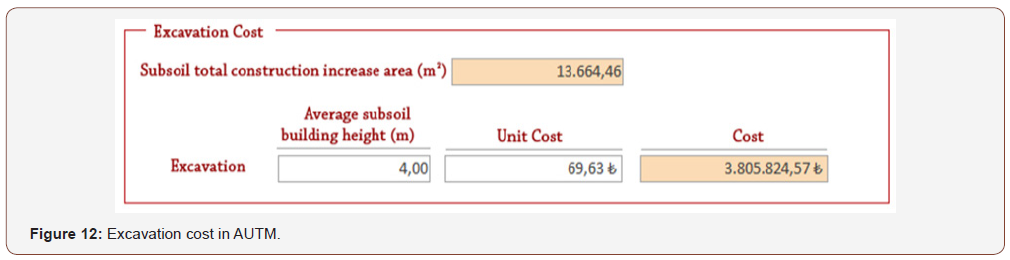##### Special Cases – Cost of special case data

The special cases title is integrated into the model considering that there may be some special conditions related to each project area. For this data, a cost coefficient and unit cost parameter are needed.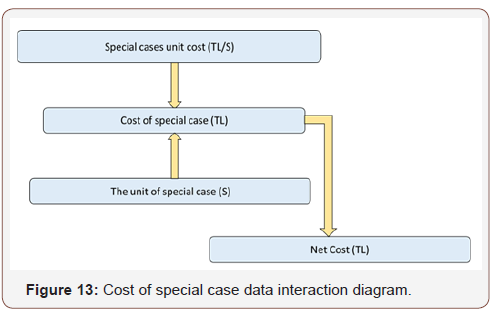The special cost belongs to cost group C (TL) as shown in the special cost data interaction diagram; is the first factor that affects at the first-degree net cost (TL) parameter from model group C (Figure 13).

The special case of the project area, which is the subject of this study, is the energy transmission line passing through the middle of the site. ETL (Energy Transmission Line) affects the project area for approximately 5,00 km. It was learned that this line will be taken to the underground as a result of the interviews with the Turkish Electricity Transmission Co. (TEIAS). It has been reported that the unit cost of ETL will be 1.500.000,00 TL/km.With the equation (9), the length of the line is calculated as 5.00km (field data) and the unit cost is 1.500.000,00 TL.

##### Appropriate total cost (TL)

As shown in the total cost data interaction diagram, the total cost (TL) of the cost group C is related to the net cost and contractor profit (Figure 14).According to the equation (10), the sum of all appropriate costs (construction costs, recreation cost, destruction cost, excavation and special case) included 25% of contractor benefits so the total urban transformation cost of the project area is calculated as 84.316.333,57 TL.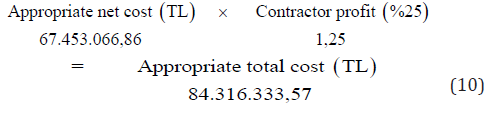#### Conclusion and Evaluation

Table 3 presents the results of the appropriate situation cost in the project area as a table. When all of the equations belonging to C group cost analysis which are created as a result of data transfer of group A and B model parameters, are examined together, the material elements of the area where transformation is needed according to AUTM are evaluated together with the results as follows:

Table 3: Cost data analysis table.As a result; the total cost of urban transformation in the risky area is calculated as 84.316.333,57 TL together with the contractor’s benefit according to the appropriate urban transformation model (AUTM), having a population of 1430, having the right to urban zoning area of 50,90%, the size of the public area up to 34,10%, the average total floor area ratio value as (1,76), with an average floor area coverage ratio value of 0.31 and limited to 6 storey of all structures. Almost 2/3 of this cost (65.84%) will be used for construction areas. The other two items, which are based on cost, 13,42% for the recreation and 11,12% of budget for the excavation were allocated. In other words, the amount of money foreseen for the construction process is 57.269.996.39 TL and it has accounted for 84.90% (approximately 4/5) of the net cost. The cost of the special case (grounding of the ETL) with the aforementioned urban transformation zone contained 11.12% of the net cost. There is also a cost of 3,98% for demolition. In the light of all these statements, it is calculated that the estimated cost value for UKDM is divided into 85% construction activity (urban infrastructure, recreation and excavation) and 15% pre-transformation preparation (demolition and special cases).

None.

#### Conflict of Interest

No conflict of interest.

Article Details
Citation
Keywords
Scroll to
Scroll to Top